Do you like the article?
Share it with others -

Use new possibilities of MetaTrader 5

12 April 2007, 13:308
15 224

### Introduction

The article deals with programmed tracking of events in the МetaТrader 4 Client Terminal, such as opening/closing/modifying orders, and is targeted at a user who has basic skills in working with the terminal and in programming in MQL 4.

### What are events and why should they be tracked?

To implement some strategies, it is not enough just to know whether there is a position opened by the Expert Advisor. Sometimes it is necessary to "catch" the moment of opening/closing/modifying a position or triggering of a pending order. There are no embedded functions in MQL4 that could solely solve this problem, but there is everything in it for creation such a tool. This is what we are going to do.

### Event Definition Principle

How can one know that an event has occurred? What is, in general, an event? Having tried to answer these questions, we will draw the following conclusion: an event is an order/open position state change. For the purposes of our task, this is, for example, changing the amount of open positions or the Stop Loss level of a position.

How can one detect that an event is taking place at the moment? It's very simple. To do this, it is necessary to memorize the value to be tracked (in our case, it is the amount of positions), and then, for example, at the next tick, compare it to the newly obtained value. Let us write a simple Expert Advisor that would inform us about changes in the amount of positions.

```int start()
{
static bool first = true;
static int pre_OrdersTotal = 0;
int _OrdersTotal = OrdersTotal();

// If this is the first launch of the Expert, we don't know the amount of orders on the previous tick.
// So just remeber it, mark that the first launch has been happened, and exit.
if ( first )
{
pre_OrdersTotal = _OrdersTotal;
first = false;
return(0);
}

// Compare the amount of positions on the previous tick with the current amount
// If it has been changed, display message
if ( _OrdersTotal > pre_OrdersTotal )
Alert( "The amount of positions increased! There were - ", pre_OrdersTotal,
", there are now - ", _OrdersTotal );

if ( _OrdersTotal < pre_OrdersTotal )
Alert( "The amount of positions decreased! There were - ", pre_OrdersTotal,
", there are now - ", _OrdersTotal );

// Memorize the amount of positions
pre_OrdersTotal = _OrdersTotal;

return(0);
}```

It is necessary to note some special features of this Expert Advisor:

• Variables first and pre_OrdersTotal are declared as static. Thus, their values are not zeroized when leaving function start(). An alternative for static variables can be global variables (declared beyond functions), but a great amount of them can cause mixing up names (one can declare a variable of the same name within the function by mistake which can cause conflicts). So we will declare all the variables in the body of the function.
• The Expert will inform about changes in amount of both open positions and pending orders (function OrdersTotal() returns their total amount).
• The Expert will not inform about triggering of a pending order since, in this case, the value of the OrdersTotal() will not change.
• The Expert will not be able to detect changes in the amount of orders at the first launch since it does not 'know' how many of them there were on the previous tick.
• the message will appear only when a new tick incomes for the symbol, in the chart of which the Expert is working. The Expert does not have any other launching events.

The last problem can be solved by placing the start function body in a loop. Thus, the checking will not take place on every tick, it will be performed at certain time intervals:

```int start()
{
static bool first = true;
static int pre_OrdersTotal = 0;
int _OrdersTotal = OrdersTotal();

// If it is the first launch of the Expert Advisor, we do not know the amount of orders on the previous tick.
// So we will just memorize it, check that the first launch has already happened, and exit.
if ( first )
{
pre_OrdersTotal = _OrdersTotal;
first = false;
return(0);
}

while ( !IsStopped() )
{
_OrdersTotal = OrdersTotal();

// Compare the amount of positions on the previous tick to the current amount.
// If it has been changed, display the message
if ( _OrdersTotal > pre_OrdersTotal )
Alert( "The amount of positions increased! There were - ", pre_OrdersTotal,
", there are now - ", _OrdersTotal );

if ( _OrdersTotal < pre_OrdersTotal )
Alert( "The amount of positions decreased! There were - ", pre_OrdersTotal,
", there are now - ", _OrdersTotal );

// Memorize the amount of positions
pre_OrdersTotal = _OrdersTotal;

Sleep(100);
}

return(0);
}```

In this above version, the message about changes in the amount of positions will appear immediately: You can check it!

### Event Filtering: Criteria

As in its current realization, our Expert Advisor will inform us about new positions appeared on all symbols. But it is more common that we only need information about changes in amounts of orders on the current symbol. Besides, orders managed by an Expert Advisor are most commonly marked with a MagicNumber. Let us filter events by these two criteria, i.e. we will inform about changes in amounts of orders for only current symbol and for only given MagicNumber.

```extern int MagicNumber = 0;

int start()
{
static bool first = true;
static int pre_OrdersTotal = 0;
int _OrdersTotal = 0, now_OrdersTotal = 0, _GetLastError = 0;

while ( !IsStopped() )
{
_OrdersTotal = OrdersTotal();
now_OrdersTotal = 0;

for ( int z = _OrdersTotal - 1; z >= 0; z -- )
{
if ( !OrderSelect( z, SELECT_BY_POS ) )
{
_GetLastError = GetLastError();
Print( "OrderSelect( ", z, ", SELECT_BY_POS ) - Error #", _GetLastError );
continue;
}
// Count the amount of orders by the current symbol and with the specified MagicNumber
if ( OrderMagicNumber() == MagicNumber &&
OrderSymbol() == Symbol() ) now_OrdersTotal ++;
}

// Display data only if this is not the first launch of the Expert
if ( !first )
{
// Compare the amount of positions on the previous tick with the current amount
// If it has changed, display the message
if ( now_OrdersTotal > pre_OrdersTotal )
Alert( Symbol(), ": amount of positions having MagicNumber ", MagicNumber,
" increased! there were - ", pre_OrdersTotal, ", there are now - ", now_OrdersTotal );

if ( now_OrdersTotal < pre_OrdersTotal )
Alert( Symbol(), ": amount of positions having MagicNumber ", MagicNumber,
" decreased! There were - ", pre_OrdersTotal, ", there are now - ", now_OrdersTotal );
}
else
{
first = false;
}
//---- Remember the amount of positions
pre_OrdersTotal = now_OrdersTotal;

Sleep(100);
}

return(0);
}```

### Refining

Finding the total amount of orders is, of course, very good, but some more detailed information is necessary sometimes – for example, "Was a buy or a sell position opened?", "Did a pending order trigger?", "Was the position closed by Stop Loss, by Take Profit, or manually?".Let us try to make the list of events to be tracked as complete as possible and divide it into groups.

1. Opening a Position
• "Market Position"
• Sell
• Pending Order
• Sell Limit
• Sell Stop
2. Order Triggering
• Sell Limit
• Sell Stop
3. Closing a Position
• "Market Position"
• Stop Loss
• Take Profit
• Manually (neither Stop Loss nor Take Profit)
• Sell
• Stop Loss
• Take Profit
• Manually
• Pending Order (deletion)
• Expiry
• Manually
• Sell Limit
• Expiry time
• Manually
• Expiry
• Manually
• Sell Stop
• Expiry
• Manually
4. Modifying a Position
• "Market Position"
• Stop Loss
• Take Profit
• Sell
• Stop Loss
• Take Profit
• Pending Order
• Open Price
• Stop Loss
• Take Profit
• Expiry
• Sell Limit
• Open Price
• Stop Loss
• Take Profit
• Expiry
• Open Price
• Stop Loss
• Take Profit
• Expiry
• Sell Stop
• Open Price
• Stop Loss
• Take Profit
• Expiry

Before we realize the algorithm, let us check whether all the above-listed events are really necessary. If we are going to create an Expert Advisor that would inform or report us about all changes in all positions, the answer is yes, all these events must be taken into consideration. But our purpose is humbler: We want to help the trading Expert Advisor to 'understand' what happens to positions it is working with. In this case, the list can be significantly shorter: position opening, placing pending orders, all modifying items and manual closing positions can be removed from the list – these events are generated by the Expert itself (they cannot happen without the Expert). Thus, this is what we have now:

1. Order Triggering
• Sell Limit
• Sell Stop
2. Position Closing
• "Market Position"
• Stop Loss
• Take Profit
• Sell
• Stop Loss
• Take Profit
• Pending Order (expiration)
• Sell Limit
• Sell Stop

As it is now, the list is much less redoubtable, so we can start writing the code. Just a little reservation should be made that there are several ways to define the position closing method (SL, TP):

• If the total amount of positions has been decreased, search in the history the most recently closed position and detect by its parameters how it had been closed, or
• memorize tickets of all open positions and then search for the 'disappeared' position by its ticket in the history.

The first way is simpler in implementation, but it can produce wrong data – if two positions are closed within the same tick, one manually and one by Stop Loss, the Expert will generate 2 identical events having found the position with the most recent close time (if the last position was closed manually, both events will be considered as manual close). The EA will not 'know' then that one of the positions has just been closed by Stop Loss.

So, in order to avoid such problems, let us write as literate code as possible.

```extern int MagicNumber = 0;

// open positions array as on the previous tick
int pre_OrdersArray[]; // [amount of positions][ticket #, position type]

int start()
{
// first launch flag
static bool first = true;
// last error code
int _GetLastError = 0;
// total amount of positions
int _OrdersTotal = 0;
// the amount of positions that meet the criteria (the current symbol and the MagicNumber),
// as on the current tick
int now_OrdersTotal = 0;
// the amount of positions that meet the criteria (the current symbol and the specified MagicNumber),
// as on the previous tick
static int pre_OrdersTotal = 0;
// open positions array as on the current tick
int now_OrdersArray[]; // [# in the list][ticket #, position type]
// the current number of the position in the array now_OrdersArray (for search)
int now_CurOrder = 0;
// the current number of the position in the array pre_OrdersArray (for search)
int pre_CurOrder = 0;

// array for storing the amount of closed positions of each type
int now_ClosedOrdersArray; // [order type][closing type]
// array for storing the amount of triggered pending orders
int now_OpenedPendingOrders; // [order type] (there are only 4 types of pending orders totally)

// temporary flags
bool OrderClosed = true, PendingOrderOpened = false;
// temporary variables
int ticket = 0, type = -1, close_type = -1;

//+------------------------------------------------------------------+
//| Infinite loop
//+------------------------------------------------------------------+
while ( !IsStopped() )
{
// memorize the total amount of positions
_OrdersTotal = OrdersTotal();
// change the open positions array size for the current amount
ArrayResize( now_OrdersArray, _OrdersTotal );
// zeroize the array
ArrayInitialize( now_OrdersArray, 0.0 );
// zeroize the amount of positions met the criteria
now_OrdersTotal = 0;

// zeroize the arrays of closed positions and triggered orders
ArrayInitialize( now_ClosedOrdersArray, 0.0 );
ArrayInitialize( now_OpenedPendingOrders, 0.0 );

//+------------------------------------------------------------------+
//| Search in all positions and write only those in the array that
//| meet the criteria
//+------------------------------------------------------------------+
for ( int z = _OrdersTotal - 1; z >= 0; z -- )
{
if ( !OrderSelect( z, SELECT_BY_POS ) )
{
_GetLastError = GetLastError();
Print( "OrderSelect( ", z, ", SELECT_BY_POS ) - Error #", _GetLastError );
continue;
}
// Count orders for the current symbol and with the specified MagicNumber
if ( OrderMagicNumber() == MagicNumber && OrderSymbol() == Symbol() )
{
now_OrdersArray[now_OrdersTotal] = OrderTicket();
now_OrdersArray[now_OrdersTotal] = OrderType();
now_OrdersTotal ++;
}
}
// change the open positions array size for the amount of positions met the criteria
ArrayResize( now_OrdersArray, now_OrdersTotal );

//+------------------------------------------------------------------+
//| Search in the list of positions on the previous tick and count
//| how many positions have been closed and pending orders triggered
//+------------------------------------------------------------------+
for ( pre_CurOrder = 0; pre_CurOrder < pre_OrdersTotal; pre_CurOrder ++ )
{
// memorize the ticket and the order type
ticket = pre_OrdersArray[pre_CurOrder];
type   = pre_OrdersArray[pre_CurOrder];
// suppose that, if it is a position, it has been closed
OrderClosed = true;
// suppose that, if it is a pending order, it has not triggered
PendingOrderOpened = false;

// search in all positions from the current list of open positions
for ( now_CurOrder = 0; now_CurOrder < now_OrdersTotal; now_CurOrder ++ )
{
// if a position with this ticket is in the list,
if ( ticket == now_OrdersArray[now_CurOrder] )
{
// the position has not been closed (the order has not been cancelled)
OrderClosed = false;

// if its type has changed,
if ( type != now_OrdersArray[now_CurOrder] )
{
// it is a pending order that has triggered
PendingOrderOpened = true;
}
break;
}
}
// if a position has not been closed (the order has not been cancelled),
if ( OrderClosed )
{
// select it
if ( !OrderSelect( ticket, SELECT_BY_TICKET ) )
{
_GetLastError = GetLastError();
Print( "OrderSelect( ", ticket, ", SELECT_BY_TICKET ) - Error #", _GetLastError );
continue;
}
// and check HOW the position has been closed (the order has been cancelled):
if ( type < 2 )
{
// Buy and Sell: 0 - manually, 1 - by SL, 2 - by TP
close_type = 0;
if ( StringFind( OrderComment(), "[sl]" ) >= 0 ) close_type = 1;
if ( StringFind( OrderComment(), "[tp]" ) >= 0 ) close_type = 2;
}
else
{
// Pending orders: 0 - manually, 1 - expiration
close_type = 0;
if ( StringFind( OrderComment(), "expiration" ) >= 0 ) close_type = 1;
}

// and write in the closed orders array that the order of type 'type'
// was cancelled as close_type
now_ClosedOrdersArray[type][close_type] ++;
continue;
}
// if a pending order has triggered,
if ( PendingOrderOpened )
{
// write in the triggered orders array that the order of type 'type' has triggered
now_OpenedPendingOrders[type-2] ++;
continue;
}
}

//+------------------------------------------------------------------+
//| Collected all necessary information - display it
//+------------------------------------------------------------------+
// if it is not the first launch of the Expert Advisor
if ( !first )
{
// search in all elements of the triggered pending orders array
for ( type = 2; type < 6; type ++ )
{
// if the element is not empty (an order of the type has triggered), display information
if ( now_OpenedPendingOrders[type-2] > 0 )
Alert( Symbol(), ": triggered ", _OrderType_str( type ), " order!" );
}

// search in all elements of the closed positions array
for ( type = 0; type < 6; type ++ )
{
for ( close_type = 0; close_type < 3; close_type ++ )
{
// if the element is not empty (the position has been closed), display information
if ( now_ClosedOrdersArray[type][close_type] > 0 ) CloseAlert( type, close_type );
}
}
}
else
{
first = false;
}

//---- save the current positions array in the previous positions array
ArrayResize( pre_OrdersArray, now_OrdersTotal );
for ( now_CurOrder = 0; now_CurOrder < now_OrdersTotal; now_CurOrder ++ )
{
pre_OrdersArray[now_CurOrder] = now_OrdersArray[now_CurOrder];
pre_OrdersArray[now_CurOrder] = now_OrdersArray[now_CurOrder];
}
pre_OrdersTotal = now_OrdersTotal;

Sleep(100);
}
return(0);
}
{
string action = "";
if ( alert_type < 2 )
{
{
case 1: action = " by StopLoss!"; break;
case 2: action = " by TakeProfit!"; break;
default: action = " manually!"; break;
}
}
else
{
{
case 1: action = " by expiration!"; break;
default: action = " manually!"; break;
}
}
}
// returns OrderType as a text
string _OrderType_str( int _OrderType )
{
switch ( _OrderType )
{
case OP_SELL:            return("Sell");
case OP_SELLLIMIT:    return("SellLimit");
case OP_SELLSTOP:        return("SellStop");
default:                    return("UnknownOrderType");
}
}```

### Integration in Expert Advisors and Usage

For convenient use of the 'event trap' from any Expert Advisor, let us locate the code in file Events.mq4 in order just to include it into EAs with the directive #include. To do so:

• form the code as a function to be called from Expert Advisors afterwards;
• remove global variable MagicNumber and add parameter of function magic (they will play the same role, we do it just in order not to block up the list of the Expert's external variables);
• add one global variable for each event – this will make their usage most comfortable (we also must insert zeroizing of these variables into the function start);
• remove the infinite loop – 'sampling' will now be made between function calls (i.e., calling a function, we will just get a list of changes compared to the preceding call of the function);
• remove Alerts, they can be then added to the Expert, if necessary;
• polish the code according to all above.

This is what we should get as a result:

```// array of open positions as it was on the previous tick
int pre_OrdersArray[]; // [amount of positions][ticket #, positions type]

// variables of events
int eventSellClosed_SL = 0, eventSellClosed_TP = 0;
int eventSellLimitDeleted_Exp = 0, eventSellStopDeleted_Exp = 0;
int eventSellLimitOpened = 0, eventSellStopOpened = 0;

void CheckEvents( int magic = 0 )
{
// flag of the first launch
static bool first = true;
// the last error code
int _GetLastError = 0;
// total amount of positions
int _OrdersTotal = OrdersTotal();
// the amount of positions met the criteria (the current symbol and the specified MagicNumber),
// as it is on the current tick
int now_OrdersTotal = 0;
// the amount of positions met the criteria as on the previous tick
static int pre_OrdersTotal = 0;
// array of open positions as of the current tick
int now_OrdersArray[]; // [# in the list][ticket #, position type]
// the current number of the position in array now_OrdersArray (for searching)
int now_CurOrder = 0;
// the current number of the position in array pre_OrdersArray (for searching)
int pre_CurOrder = 0;

// array for storing the amount of closed positions of each type
int now_ClosedOrdersArray; // [order type][closing type]
// array for storing the amount of triggered pending orders
int now_OpenedPendingOrders; // [order type]

// temporary flags
bool OrderClosed = true, PendingOrderOpened = false;
// temporary variables
int ticket = 0, type = -1, close_type = -1;

//zeroize the variables of events
eventSellClosed_SL = 0; eventSellClosed_TP = 0;
eventSellLimitDeleted_Exp = 0; eventSellStopDeleted_Exp = 0;
eventSellLimitOpened = 0; eventSellStopOpened = 0;

// change the open positions array size for the current amount
ArrayResize( now_OrdersArray, MathMax( _OrdersTotal, 1 ) );
// zeroize the array
ArrayInitialize( now_OrdersArray, 0.0 );

// zeroize arrays of closed positions and triggered orders
ArrayInitialize( now_ClosedOrdersArray, 0.0 );
ArrayInitialize( now_OpenedPendingOrders, 0.0 );

//+------------------------------------------------------------------+
//| Search in all positions and write in the array only those
//| meeting the criteria
//+------------------------------------------------------------------+
for ( int z = _OrdersTotal - 1; z >= 0; z -- )
{
if ( !OrderSelect( z, SELECT_BY_POS ) )
{
_GetLastError = GetLastError();
Print( "OrderSelect( ", z, ", SELECT_BY_POS ) - Error #", _GetLastError );
continue;
}
// Count the amount of orders on the current symbol with the specified MagicNumber
if ( OrderMagicNumber() == magic && OrderSymbol() == Symbol() )
{
now_OrdersArray[now_OrdersTotal] = OrderTicket();
now_OrdersArray[now_OrdersTotal] = OrderType();
now_OrdersTotal ++;
}
}
// change the open positions array size for the amount of positions meeting the criteria
ArrayResize( now_OrdersArray, MathMax( now_OrdersTotal, 1 ) );

//+-------------------------------------------------------------------------------------------------+
//| Search in the list of the previous tick positions and count how many positions have been closed
//| and pending orders triggered
//+-------------------------------------------------------------------------------------------------+
for ( pre_CurOrder = 0; pre_CurOrder < pre_OrdersTotal; pre_CurOrder ++ )
{
// memorize the ticket number and the order type
ticket = pre_OrdersArray[pre_CurOrder];
type   = pre_OrdersArray[pre_CurOrder];
// assume that, if it is a position, it has been closed
OrderClosed = true;
// assume that, if it is a pending order, it has not triggered
PendingOrderOpened = false;

// search in all positions from the current list of open positions
for ( now_CurOrder = 0; now_CurOrder < now_OrdersTotal; now_CurOrder ++ )
{
// if there is a position with such a ticket number in the list,
if ( ticket == now_OrdersArray[now_CurOrder] )
{
// it means that the position has not been closed (the order has not been cancelled)
OrderClosed = false;

// if its type has changed,
if ( type != now_OrdersArray[now_CurOrder] )
{
// it means that it was a pending order and it triggered
PendingOrderOpened = true;
}
break;
}
}
// if a position has been closed (an order has been cancelled),
if ( OrderClosed )
{
// select it
if ( !OrderSelect( ticket, SELECT_BY_TICKET ) )
{
_GetLastError = GetLastError();
Print( "OrderSelect( ", ticket, ", SELECT_BY_TICKET ) - Error #", _GetLastError );
continue;
}
// and check HOW the position has been closed (the order has been cancelled):
if ( type < 2 )
{
// Buy and Sell: 0 - manually, 1 - by SL, 2 - by TP
close_type = 0;
if ( StringFind( OrderComment(), "[sl]" ) >= 0 ) close_type = 1;
if ( StringFind( OrderComment(), "[tp]" ) >= 0 ) close_type = 2;
}
else
{
// Pending orders: 0 - manually, 1 - expiration
close_type = 0;
if ( StringFind( OrderComment(), "expiration" ) >= 0 ) close_type = 1;
}

// and write in the closed orders array that the order of the type 'type'
// was closed by close_type
now_ClosedOrdersArray[type][close_type] ++;
continue;
}
// if a pending order has triggered,
if ( PendingOrderOpened )
{
// write in the triggered orders array that the order of type 'type' triggered
now_OpenedPendingOrders[type-2] ++;
continue;
}
}

//+--------------------------------------------------------------------------------------------------+
//| All necessary information has been collected - assign necessary values to the variables of events
//+--------------------------------------------------------------------------------------------------+
// if it is not the first launch of the Expert Advisor
if ( !first )
{
// search in all elements of the triggered pending orders array
for ( type = 2; type < 6; type ++ )
{
// if the element is not empty (an order of the type has not triggered), change the variable value
if ( now_OpenedPendingOrders[type-2] > 0 )
SetOpenEvent( type );
}

// search in all elements of the closed positions array
for ( type = 0; type < 6; type ++ )
{
for ( close_type = 0; close_type < 3; close_type ++ )
{
// if the element is not empty (a position has been closed), change the variable value
if ( now_ClosedOrdersArray[type][close_type] > 0 )
SetCloseEvent( type, close_type );
}
}
}
else
{
first = false;
}

//---- save the current positions array in the previous positions array
ArrayResize( pre_OrdersArray, MathMax( now_OrdersTotal, 1 ) );
for ( now_CurOrder = 0; now_CurOrder < now_OrdersTotal; now_CurOrder ++ )
{
pre_OrdersArray[now_CurOrder] = now_OrdersArray[now_CurOrder];
pre_OrdersArray[now_CurOrder] = now_OrdersArray[now_CurOrder];
}
pre_OrdersTotal = now_OrdersTotal;
}
void SetOpenEvent( int SetOpenEvent_type )
{
switch ( SetOpenEvent_type )
{
case OP_SELLLIMIT: eventSellLimitOpened ++; return(0);
case OP_SELLSTOP: eventSellStopOpened ++; return(0);
}
}
void SetCloseEvent( int SetCloseEvent_type, int SetCloseEvent_close_type )
{
switch ( SetCloseEvent_type )
{
{
if ( SetCloseEvent_close_type == 1 ) eventBuyClosed_SL ++;
if ( SetCloseEvent_close_type == 2 ) eventBuyClosed_TP ++;
return(0);
}
case OP_SELL:
{
if ( SetCloseEvent_close_type == 1 ) eventSellClosed_SL ++;
if ( SetCloseEvent_close_type == 2 ) eventSellClosed_TP ++;
return(0);
}
{
if ( SetCloseEvent_close_type == 1 ) eventBuyLimitDeleted_Exp ++;
return(0);
}
{
if ( SetCloseEvent_close_type == 1 ) eventBuyStopDeleted_Exp ++;
return(0);
}
case OP_SELLLIMIT:
{
if ( SetCloseEvent_close_type == 1 ) eventSellLimitDeleted_Exp ++;
return(0);
}
case OP_SELLSTOP:

{
if ( SetCloseEvent_close_type == 1 ) eventSellStopDeleted_Exp ++;
return(0);
}
}
}```

Events can now be tracked from any Expert Advisor just by enabling a library. An example of such Expert Advisor (EventsExpert.mq4) is given below:

```extern int MagicNumber = 0;

#include <Events.mq4>

int start()
{
CheckEvents( MagicNumber );

if ( eventBuyClosed_SL > 0 )

if ( eventBuyClosed_TP > 0 )

eventSellLimitOpened > 0 || eventSellStopOpened > 0 )
Alert( Symbol(), ": pending order triggered!" );
return(0);
}```

### 6. Conclusion

In this article, we considered the ways of programmed tracking events in the МetaТrader 4 by means of MQL4. We divided all events into 3 groups and filtered them according to the predefined criteria. We also created a library that allows easy tracking of some events from any Expert Advisor.

Function CheckEvents() can be completed (or used as a template) to track other events that have not been considered in the article.

Translated from Russian by MetaQuotes Software Corp.
Original article: https://www.mql5.com/ru/articles/1399

Attached files |
Events.mq4 (8.62 KB)
EventsExpert.mq4 (0.93 KB)
Last comments | Go to discussion (8)| 18 Nov 2008 at 06:06
ukt:

Interesting article Andre. Your method of obtaining closed reason is by inspection of order comment.

Is this 100% generic solution that will apply to brokers where I can trade using EA's?

It is better to analyse a OrderClosePrice():

```if ( MathAbs( OrderClosePrice() - OrderStopLoss() ) < Point ) // closed by SL
if ( MathAbs( OrderClosePrice() - OrderTakeProfit() ) < Point ) // closed by TP```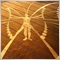| 8 Apr 2010 at 11:09
How can I add and alert when I open a buy or sell position? Is it possible? Thanks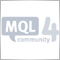| 17 Aug 2010 at 17:07

Hi, is it possible to retrieve the Order information (i.e. ticket number, closed price ...) when an event is triggered?

```int start()
{
CheckEvents( MagicNumber );
OrderInfoObj orderInfo;
if ( (orderInfo = eventBuyClosed_SL) > 0 )
Alert( Symbol(), ": Buy position was closed by StopLoss!", orderInfo.ticket, orderInfo.closedPrice ... );
....
return(0); ```

}| 31 Oct 2017 at 08:08

Awsome article, Thank you sir!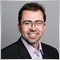| 17 Jun 2018 at 03:50
Very good article.  But I noticed when I tried to use it inside a code that if I open a Sell Stop and then the Sell Stop is activated and becomes a Sell and there is a Stop Loss there is no event triggered.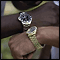Synchronization of Expert Advisors, Scripts and Indicators

The article considers the necessity and general principles of developing a bundled program that would contain both an Expert Advisor, a script and an indicator.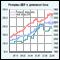Poll: Traders’ Estimate of the Mobile Terminal

Unfortunately, there are no clear projections available at this moment about the future of the mobile trading. However, there are a lot of speculations surrounding this matter. In our attempt to resolve this ambiguity we decided to conduct a survey among traders to find out their opinion about our mobile terminals. Through the efforts of this survey, we have managed to established a clear picture of what our clients currently think about the product as well as their requests and wishes in future developments of our mobile terminals.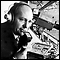Expert System 'Commentator'. Practical Use of Embedded Indicators in an MQL4 Program

The article describes the use of technical indicators in programming on MQL4.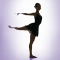Pivot Points Helping to Define Market Trends

Pivot point is a line in the price chart that shows the further trend of a currency pair. If the price is above this line, it tends to grow. If the price is below this line, accordingly, it tends to fall.